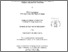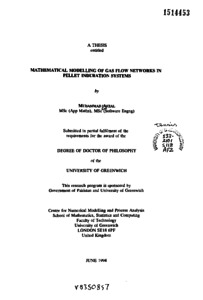# Mathematical modelling of gas flow networks in pellet induratlon systems

Afzal, Muhammad (1994) Mathematical modelling of gas flow networks in pellet induratlon systems. PhD thesis, University of Greenwich.Preview
PDF
Muhammad Afzal 1994.pdf - Published Version

## Abstract

The objective of this research is to develop a simulation software tool, GASFLO, which should evaluate pressure, flow and temperature distributions of process gas in pellet induration system networks. Pellet induration systems are complex industrial systems composed of heterogenous components. The magnitude of gas through leaks i.e. the air entering or leaving the system from the points other than the known exits, is substantial and it adversely effects the performance of induration process. These leaks are very difficult to measure because of the hostile environment in the plant. The modelling of such industrial systems requires a notable amount of experimentation so the tool has been designed to enable the user modeller to change the component models and solution algorithms easily.

The conventional methods for flow network simulation are based on process centred approach, mostly composed of homogeneous components. For ease of computation, the non-pipe elements are modelled with an approximate linear or non-linear generic equation, whose coefficients can simulate different states of the element. The resulting set of non-linear equations is linearised and solved simultaneously using some iterative method. By contrast, GASFLO is based on device centred or unit based approach, and uses a two level hierarchical solution algorithm. The pellet induration system network is first idealised into a connected graph of streams (sets of serially connected components) and nodes. At the top or coordination level the flow and pressure distributions satisfying the Kirchhoff's laws are evaluated for the connected graph. At the lower or component level the exact mathematical models of components ale computed, in order of their occurrence in respective streams, using coordination variables as parameters. The converged flows are used for the temperature computation. The solution algorithm requires partitioning of the connected graph into forest and coforest structures, for which secondary algorithms have been developed using specific heuristics relevant to the pellet induration systems. The rigorous application of software engineering techniques for the design and implementation of software, enabled the resolution of the complexity of the modelled system, embedded the characteristics of 'quality software' into the resulting code and benefits from object orientation, even though it is implemented in standard FORTRAN 77.

GASFLO predicted results are in a good agreement with the measured results, it has been validated for a real life pellet induration system. It has been applied to simulate several practical scenarios, like addition of extra wind boxes to the zones and to determine how the plant production can be increased by certain ratio, such simulations were not feasible otherwise. GASFLO takes less than a minute to simulate a real-life pellet induration system on a 486 PC. The combined simulation with an other software tool, INDSYS, which evaluates the heat distribution in the solids, is also feasible.

Item Type: Thesis (PhD) uk.bl.ethos.530894 mathematical modelling, simulation software, Q Science > QA Mathematics School of Computing & Mathematical SciencesSchool of Computing & Mathematical Sciences > Centre for Numerical Modelling & Process Analysis 14 Oct 2016 09:15 http://gala.gre.ac.uk/id/eprint/5700View Item